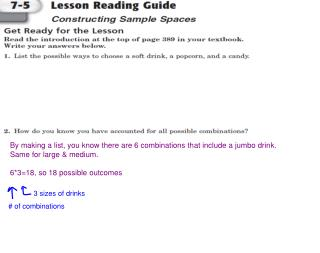DownloadDownload Presentation# of combinations

# of combinations

Télécharger la présentation# of combinations

- - - - - - - - - - - - - - - - - - - - - - - - - - - E N D - - - - - - - - - - - - - - - - - - - - - - - - - - -
Presentation Transcript

1. By making a list, you know there are 6 combinations that include a jumbo drink. Same for large & medium. 6*3=18, so 18 possible outcomes 3 sizes of drinks # of combinations

2. Finding Sample Spaces with Tree Diagrams Asample space is a list of all possible outcomes of an experiment. If we rolled a die, the sample space would be 1,2,3,4,5, and 6. How can we organize a sample space if more than one event occurs?

3. Tree Diagram Atree diagram is a organizational tool we can use to find a sample space. Kardashian is going to have two children. What are all the possible  combinations of boys and girls she can get? (sample space)

4. What shall I wear? Jennifer has 3 skirts and 2 tops. How many different outfits could she wear?

5. Make an organized list Skirt Top Red Pink Skirt Top Brown Red Red Pink Red Brown Blue Pink Blue Pink Blue Brown Blue Brown Green Pink Green Pink Green Brown Green Brown

6. Make a tree diagram P P P R B R B B P B B B P P G B B There are 6 outfit choices.

7. How many outfit choices now?

8. Solution There are 12 outfit choices.

9. Calculating the total number of outcomes

10. Find the total number of outcomes Flip a coin and roll a dice 6 x 2 = 12 Spin the spinner, roll a dice 6 x 8 = 48 Roll two six-sided dice 6 x 6 = 36

11. How many different dinner choices are there? Plates Desserts Sides Onion Soup Steak tips Ice Cream Greek salad Canolis Chicken Pie French Fries Lasagne Cheeseburger Burritos You must choose 1 side, 1 plate and 1 dessert 3 x 5 x 2 = 30

12. What are all of the possible outcomes when you flip two coins? Outcomes H T You may not need all the boxes! Make a tree diagram and an organized list

13. Sample space Outcomes H H T H T T 4 (2 x 2) How many possible outcomes are there? How many outcomes include flipping at least one head? 3 What is the probability of flipping at least one head? 3 (0.75 or 75%) 4

14. What's the probability of spinning a green and an odd number? Red Blue 2 1 4 3 Green

15. Solution 1 1 2 2 4 3 Red 3 4 ? How many outcomes? 12 1 2 Blue How many outcomes with green and an odd number? ? 2 3 4 1 Probability of green and an odd number occurring? 2 Green 2 1 3 ? ? or 12 6 4

16. Independent events 2 1 4 3 No If you spin a green on Spinner 1, does this affect what  you spin on Spinner 2? Independent events: the outcome of one event does not  affect the outcome of another. If two events are independent,  to find the probability of them both occurring, multiply the  probabilities of the individual events occurring. For example, probability of spinning a blue and spinning a 2: 1 4 1 12 1 P(Blue and 2) = = 3

17. Flip a coin and roll a dice What is the probability of flipping a tail and rolling a 5? ? Total # outcomes 12 Click to reveal the total number of  outcomes and then use the Fill Tool  to shade the cells where the outcome  of the coin flip is a tail and the outcome of the dice roll is a 5. = P(Tail and 5) = ( 1 1 1 ( P(Tail and 5) = 12 2 6

18. Flip a coin and roll a dice What is the probability of flipping a head and rolling a factor of 6? ? Total # outcomes 12 Click to reveal the total number of  outcomes and then use the Fill Tool to  shade the cells where the outcome of  the coin flip is a head and the outcome of the dice roll is a factor of 6. = P(Head and Factor of 6) = ( 1 1 4 ( P (H and F6) = 3 2 6

19. Picking a ball from each jar What's the probability of picking a red ball  from jar 1 and a green ball from jar 2? 1 1 1 2 = = 4 5 10 2 What's the probability of picking a yellow  ball from jar 1 and a yellow ball from jar 2? 1 2 3 3 = = 5 4 10 2

20. True or false? The first four questions of the math test are "True/False" type questions. Jason has not studied for the test and so he  guesses the answers. What is the probability he guesses the  correct answer for all four questions? — — P(False) = P(True) = — P(all answers are True) = — — — — 1 2 1 2 1 2 1 2 1 16 or 0.0625 =

21. What is the sample space, if we rolled the die and spun a spinner that  had the colors orange, purple, green and red?

22. http://studyjams.scholastic.com/studyjams/jams/math/probability/tree-diagrams.htmhttp://studyjams.scholastic.com/studyjams/jams/math/probability/tree-diagrams.htm

23. OUTCOME FLAVOR Set of all possible outcomes = sample space ACG, AGC, CAG, CGA, GAC, GCA. CONE Outcome column shows sample space 3 types of crust 6 toppings

24. as a fraction as a percent as a decimal